# Setup for 5 Player a Game of Food Chain Magnate

Originally generated on 1/11/2019 1:03:24 AM
A direct link to this setup is http://www.VentersConsulting.com/BoardgameHelpers/FoodChainMagnateGenerator.aspx?LoadGame=\$2a\$04\$McP7MVIpMP4v8IVLUI0Ayu

## Player Setup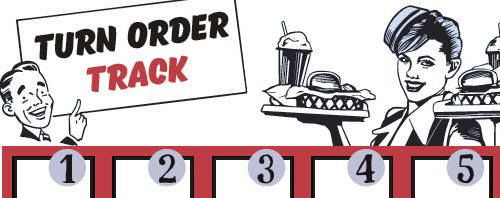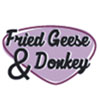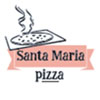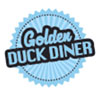## Other Setup

Bank starts with \$250.00
Number of 1x Employee cards used = 3

## Map (Dehydration Land)

Click here to view Map Tile Lettering Key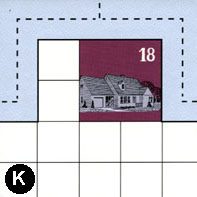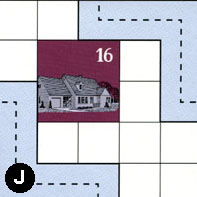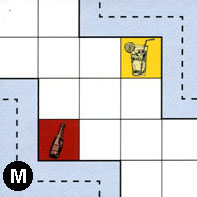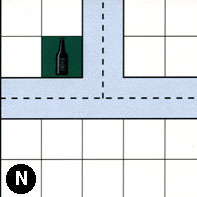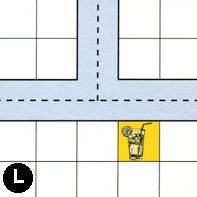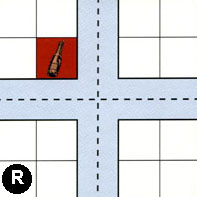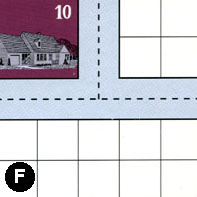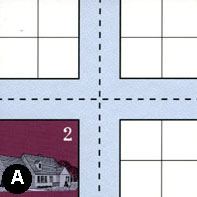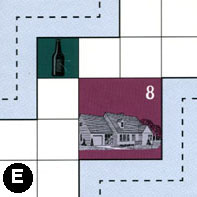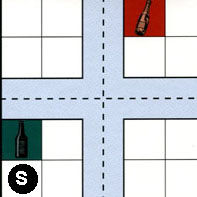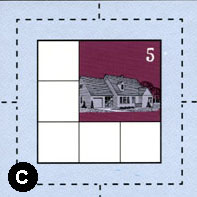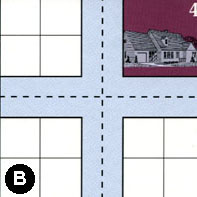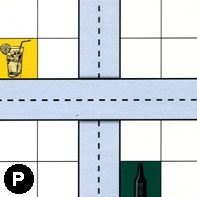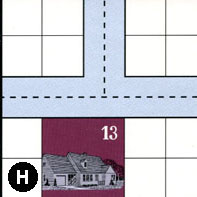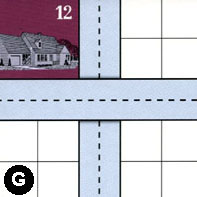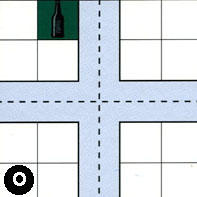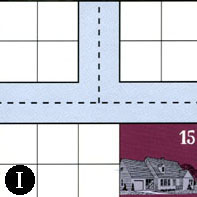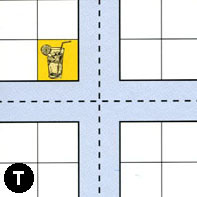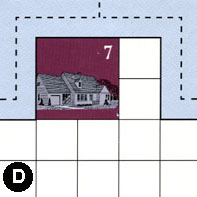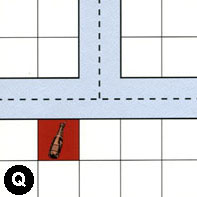### Map Stats

Map Option = Dehydration Land
Total Number of Tiles = 20
Total Number of Starting Houses = 11
Total Number of Beer Spots = 5
Total Number of Soda Spots = 4
Total Number of Lemonade Spots = 4
Total Number of Drink Spots = 13
Number of independent path systems = 3
Number of independent neighborhoods = 19

Path System 1
Size = Medium
Number of Tiles = 18
Number of Paths = 64
Contains Loop(s) = No
Number of Starting Houses = 11
Starting Houses Pct of Map (%) = 100
Number of Beer Spots = 4
Number of Soda Spots = 2
Number of Lemonade Spots = 3
Number of Drink Spots = 9
Drink Spots Pct of Map (%) = 69
Tile Ids = A, B, C, D, E, F, G, H, I, J, K, L, M, N, O, P, R, T
Paths = KWE, RWE, CWN, BWN, BWE, FWE, JEN, FWN, AWN, MWS, JWS, ANE, EWN, NWE, NWN, LWN, LNE, LWE, NNE, AWE, FNE, BNE, CNE, OWN, IWE, TWE, TWN, TNE, IWN, INE, ONE, OWE, CWE, RWN, RNE, KWN, KNE, RES, RWS, CWS, CNS, BNS, PNS, HWE, GNS, HWN, DWN, DNE, DWE, HNE, BWS, BES, CES, OWS, ONS, OES, RNS, TES, PWE, AWS, ANS, AES, TWS, TNS What do these mean?
Starting House Ids = 0, 2, 4, 5, 7, 10, 12, 13, 15, 16, 18
Path System 2
Size = Tiny
Number of Tiles = 1
Number of Paths = 1
Contains Loop(s) = No
Number of Starting Houses = 1
Starting Houses Pct of Map (%) = 9
Number of Beer Spots = 0
Number of Soda Spots = 0
Number of Lemonade Spots = 1
Number of Drink Spots = 1
Drink Spots Pct of Map (%) = 8
Tile Ids = M
Paths = MEN What do these mean?
Starting House Ids = 0
Path System 3
Size = Medium
Number of Tiles = 4
Number of Paths = 11
Contains Loop(s) = No
Number of Starting Houses = 4
Starting Houses Pct of Map (%) = 36
Number of Beer Spots = 1
Number of Soda Spots = 2
Number of Lemonade Spots = 0
Number of Drink Spots = 3
Drink Spots Pct of Map (%) = 23
Tile Ids = E, G, Q, S
Paths = SWE, EES, SWN, SNE, SES, GWE, QWN, QNE, QWE, SWS, SNS What do these mean?
Starting House Ids = 0, 8, 12, 13

Neighborhood 1
Total Size = Large
Number of Total Spaces = 61
Number of Empty Spaces (for new houses & gardens) = 45
Number of Beer Spots = 0
Number of Soda Spots = 0
Number of Lemonade Spots = 0
Number of Drink Spots = 0
Number of Starting Houses = 4
Starting Houses Pct of Map (%) = 36
Tile Ids = A, B, F, J, K, R
Starting House Ids = 4, 10, 16, 18
Neighborhood 2
Total Size = Large
Number of Total Spaces = 29
Number of Empty Spaces (for new houses & gardens) = 27
Number of Beer Spots = 0
Number of Soda Spots = 1
Number of Lemonade Spots = 1
Number of Drink Spots = 2
Number of Starting Houses = 0
Starting Houses Pct of Map (%) = 0
Tile Ids = A, M, N
Neighborhood 3
Total Size = Medium
Number of Total Spaces = 8
Number of Empty Spaces (for new houses & gardens) = 7
Number of Beer Spots = 1
Number of Soda Spots = 0
Number of Lemonade Spots = 0
Number of Drink Spots = 1
Number of Starting Houses = 0
Starting Houses Pct of Map (%) = 0
Tile Ids = L, N
Neighborhood 4
Total Size = Small
Number of Total Spaces = 4
Number of Empty Spaces (for new houses & gardens) = 4
Number of Beer Spots = 0
Number of Soda Spots = 0
Number of Lemonade Spots = 0
Number of Drink Spots = 0
Number of Starting Houses = 0
Starting Houses Pct of Map (%) = 0
Tile Ids = L
Neighborhood 5
Total Size = Large
Number of Total Spaces = 63
Number of Empty Spaces (for new houses & gardens) = 50
Number of Beer Spots = 2
Number of Soda Spots = 1
Number of Lemonade Spots = 2
Number of Drink Spots = 5
Number of Starting Houses = 2
Starting Houses Pct of Map (%) = 18
Tile Ids = A, E, G, H, L, N, P, S
Starting House Ids = 8, 13
Neighborhood 6
Total Size = Small
Number of Total Spaces = 4
Number of Empty Spaces (for new houses & gardens) = 4
Number of Beer Spots = 0
Number of Soda Spots = 0
Number of Lemonade Spots = 0
Number of Drink Spots = 0
Number of Starting Houses = 0
Starting Houses Pct of Map (%) = 0
Tile Ids = R
Neighborhood 7
Total Size = Small
Number of Total Spaces = 4
Number of Empty Spaces (for new houses & gardens) = 3
Number of Beer Spots = 0
Number of Soda Spots = 1
Number of Lemonade Spots = 0
Number of Drink Spots = 1
Number of Starting Houses = 0
Starting Houses Pct of Map (%) = 0
Tile Ids = R
Neighborhood 8
Total Size = Medium
Number of Total Spaces = 16
Number of Empty Spaces (for new houses & gardens) = 12
Number of Beer Spots = 0
Number of Soda Spots = 0
Number of Lemonade Spots = 0
Number of Drink Spots = 0
Number of Starting Houses = 1
Starting Houses Pct of Map (%) = 9
Tile Ids = A, B, F, P
Starting House Ids = 2
Neighborhood 9
Total Size = Medium
Number of Total Spaces = 8
Number of Empty Spaces (for new houses & gardens) = 8
Number of Beer Spots = 0
Number of Soda Spots = 0
Number of Lemonade Spots = 0
Number of Drink Spots = 0
Number of Starting Houses = 0
Starting Houses Pct of Map (%) = 0
Tile Ids = G, S
Neighborhood 10
Total Size = Medium
Number of Total Spaces = 9
Number of Empty Spaces (for new houses & gardens) = 5
Number of Beer Spots = 0
Number of Soda Spots = 0
Number of Lemonade Spots = 0
Number of Drink Spots = 0
Number of Starting Houses = 1
Starting Houses Pct of Map (%) = 9
Tile Ids = C
Starting House Ids = 5
Neighborhood 11
Total Size = Large
Number of Total Spaces = 30
Number of Empty Spaces (for new houses & gardens) = 24
Number of Beer Spots = 2
Number of Soda Spots = 0
Number of Lemonade Spots = 0
Number of Drink Spots = 2
Number of Starting Houses = 1
Starting Houses Pct of Map (%) = 9
Tile Ids = B, I, O, P, T
Starting House Ids = 15
Neighborhood 12
Total Size = Large
Number of Total Spaces = 32
Number of Empty Spaces (for new houses & gardens) = 27
Number of Beer Spots = 0
Number of Soda Spots = 0
Number of Lemonade Spots = 1
Number of Drink Spots = 1
Number of Starting Houses = 1
Starting Houses Pct of Map (%) = 9
Tile Ids = D, H, P, T
Starting House Ids = 7
Neighborhood 13
Total Size = Medium
Number of Total Spaces = 18
Number of Empty Spaces (for new houses & gardens) = 13
Number of Beer Spots = 0
Number of Soda Spots = 1
Number of Lemonade Spots = 0
Number of Drink Spots = 1
Number of Starting Houses = 1
Starting Houses Pct of Map (%) = 9
Tile Ids = G, H, Q
Starting House Ids = 12
Neighborhood 14
Total Size = Medium
Number of Total Spaces = 8
Number of Empty Spaces (for new houses & gardens) = 8
Number of Beer Spots = 0
Number of Soda Spots = 0
Number of Lemonade Spots = 0
Number of Drink Spots = 0
Number of Starting Houses = 0
Starting Houses Pct of Map (%) = 0
Tile Ids = G, Q
Neighborhood 15
Total Size = Small
Number of Total Spaces = 4
Number of Empty Spaces (for new houses & gardens) = 4
Number of Beer Spots = 0
Number of Soda Spots = 0
Number of Lemonade Spots = 0
Number of Drink Spots = 0
Number of Starting Houses = 0
Starting Houses Pct of Map (%) = 0
Tile Ids = O
Neighborhood 16
Total Size = Small
Number of Total Spaces = 4
Number of Empty Spaces (for new houses & gardens) = 4
Number of Beer Spots = 0
Number of Soda Spots = 0
Number of Lemonade Spots = 0
Number of Drink Spots = 0
Number of Starting Houses = 0
Starting Houses Pct of Map (%) = 0
Tile Ids = O
Neighborhood 17
Total Size = Medium
Number of Total Spaces = 8
Number of Empty Spaces (for new houses & gardens) = 8
Number of Beer Spots = 0
Number of Soda Spots = 0
Number of Lemonade Spots = 0
Number of Drink Spots = 0
Number of Starting Houses = 0
Starting Houses Pct of Map (%) = 0
Tile Ids = I, O
Neighborhood 18
Total Size = Medium
Number of Total Spaces = 8
Number of Empty Spaces (for new houses & gardens) = 8
Number of Beer Spots = 0
Number of Soda Spots = 0
Number of Lemonade Spots = 0
Number of Drink Spots = 0
Number of Starting Houses = 0
Starting Houses Pct of Map (%) = 0
Tile Ids = I, T
Neighborhood 19
Total Size = Small
Number of Total Spaces = 4
Number of Empty Spaces (for new houses & gardens) = 4
Number of Beer Spots = 0
Number of Soda Spots = 0
Number of Lemonade Spots = 0
Number of Drink Spots = 0
Number of Starting Houses = 0
Starting Houses Pct of Map (%) = 0
Tile Ids = Q

v8.3.2